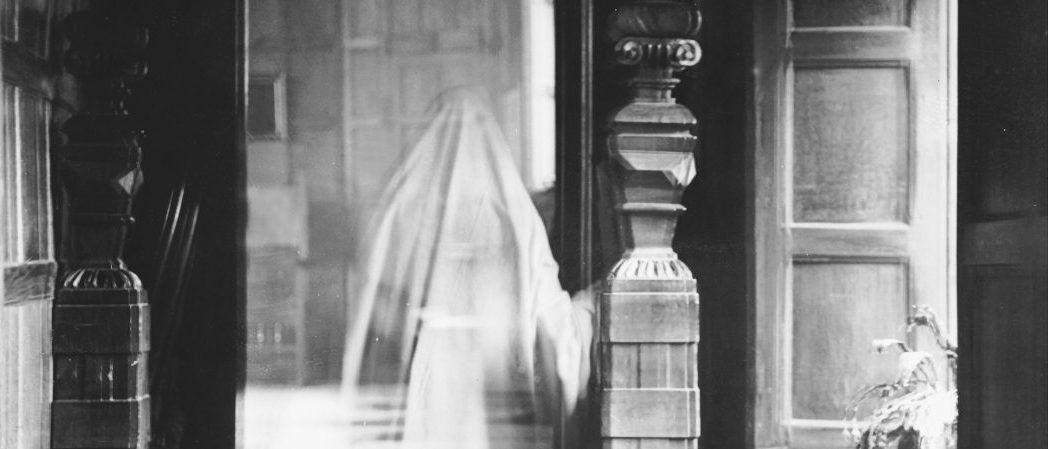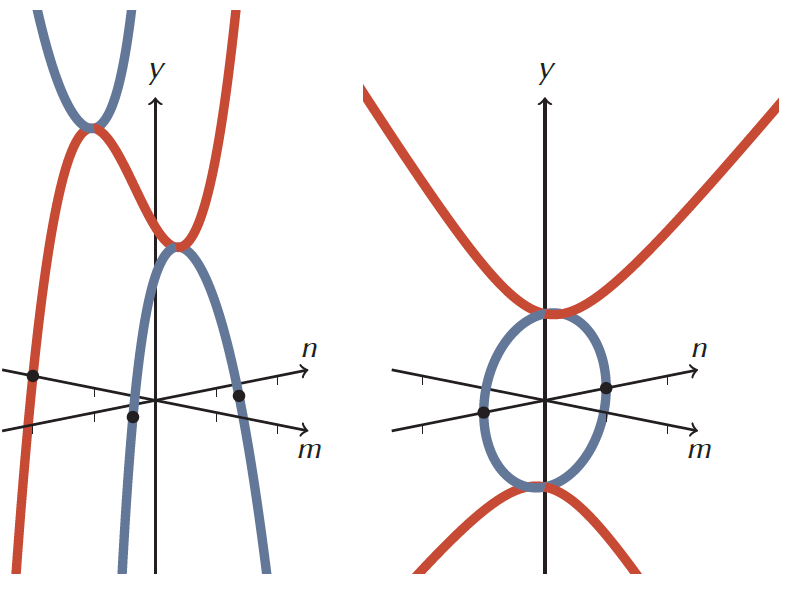# The phantom parabolaNational Archives UK

I originally wrote this piece as my semi-final entry for the Big Internet Math-Off, an online competition run by the Aperiodical. The knock-out tournament saw $2^4$ mathematicians go head-to-head in presenting ideas they found particularly interesting. Each round readers voted for their favourite of $2^1$ entries, with the winner advancing to the next round, until only $2^0$ participant emerged victorious.

The audience was given the brief to vote for the entry that made them ‘do the loudest “aha!”‘ This ‘aha!’ success criterion seemed entirely fitting since our response to mathematics is often exactly this; involuntary, emotional and beyond the reach of words. As such, when asked ‘why do you like mathematics?’, I always feel words cannot fully capture quite what it is to find something mathematically satisfying, or mathematically beautiful.

But mathematics itself is the opposite; using maths we can convey huge ideas, completely, and efficiently. When we look at things mathematically it is like we are casting a perfect light on to the world that allows us to see the very structure and essence of things.

I chose to write about the phantom parabola because it demonstrates this power; by using mathematics (in a simple, ‘aha!’-inducing way) we reveal something that was otherwise hidden. I introduce the phantom parabola as a ghost story, in a blatant clickbait-esque attempt to make people read on and vote. But as this is a story about invisible mathematics that genuinely gives me the goose bumps, as well as an ‘aha!’, I think my claim is justified.

#### The phantom parabola

This is the closest you’ll ever get to a maths ghost story—meet the phantom parabola that hangs, unnoticed, perpendicular to every quadratic and allows us to visualise complex solutions.

Roots of quadratics with real coefficients come in one of three situations; either the parabola intersects the $x$-axis twice giving us two real solutions, or it turns on the $x$-axis making one repeated real solution, or it never reaches the $x$-axis. In this final situation, we know that instead of real solutions we have a (conjugate) pair of complex solutions that cannot be represented on our real $x$-axis.

It seems incomplete to not have the corresponding visualisation of complex solutions. This, after all, is an area of mathematics that showcases the powerful and elegant unity that exists between the algebraic and graphical representations, as dreamt up by Descartes all those years ago. But then it breaks down when those solutions are complex. How dissatisfying!Enter the phantom parabola! The complex solutions of the quadratic sit on this phantom which hangs ‘upside down’ and at right angles to the original, with the two parabolas meeting at their extremes.

And we can plot the phantom parabola if we represent the values not on a one-dimensional $x$-axis, but on a two-dimensional $x$-plane, that has a real axis and an imaginary axis. The $y$-axis is then perpendicular to this plane. By following the steps below, we can work out what the phantom looks like:

• We are finding the roots of the quadratic $y=ax\hspace{1pt}^2+c$ where the constants are real. To find the roots of a general quadratic, write it in the form $y=a(x-b)^2+c$ and set $v=x-b$. You can then follow the rest of these steps with $v$ in the place of $x$.
• Since $x$ is complex, let $x=m+n\hspace{1pt} i$, where $m$ and $n$ are the axes of the complex $x$-plane.• We will now look for the values of $x$ when $y=0$.
• $y$ is real and therefore $x$ must either have only a real component or only an imaginary component (which when squared would become real).
• If $x$ is real, then $n=0$ and $y=am\hspace{.5pt}^2+c$. (This quadratic is the original one.)
• If $x$ has only an imaginary component, then $m=0$ and $y=a(\hspace{-1pt}n\hspace{1pt} i)^2+c$. This simplifies to $y=-an\hspace{.5pt}^2+c$. This is the phantom parabola!

We can understand that the parabola and its phantom are perpendicular to each other because the original only varies over the real $m$ dimension, whilst the phantom is fixed to take only one value on the real $m$-axis but can take any value on the imaginary $n$-axis. Equally, the phantom is an inversion of the original because its coefficient $a$ has been multiplied by $i^2$.

The plot below shows a specific parabola and its phantom.The parabola $y = m^2 + 2$ (red) and its phantom $y = -n^2 +2$ (blue) viewed from three different angles. The roots at $n = \pm \sqrt{2}$ (or $x = \pm \sqrt{2} i$) are shown.

Finally we glimpse the complex solutions! The quadratic in this case ($y=x\hspace{1pt}^2+2$) has roots at $x=\pm\sqrt2 i$ which can be found at the points marked above where the phantom curve crosses the plane with equation $y=0$ (the complex $x$-plane). This is of course analogous to where real solutions of a quadratic appear on a 2D plot.

Every parabola has a phantom and if we plot both it and the original parabola we will always be able to visualise the two roots of the quadratic.

In the case when the two roots are real and distinct the phantom turns before the complex $x$-plane, with the original curve crossing the plane instead.

And in the case when we have what we call a repeated real root? Well, how befitting is it that in this situation we have two curves, the original and the phantom, both touching the $x$-plane in the same place but from opposite directions?Left: the parabola $y = x^2 – 0.4$ has two real roots; its phantom is below the $x$-plane. Right: the parabola $y = x^2$ has a repeated root; it meets its phantom at this root.

At geogebra.org/m/wuwekaxy, you can find an interactive GeoGebra plot of the general quadratic and its phantom. Vary the constants $a$ and $c$ and see for yourself the two solutions of the quadratic in each different situation. Never again must we take it blindly on trust that every quadratic has two solutions!Left: the cubic $y = x^3 + x^2 – x +2$ and its phantom. Right: the hyperbola $y^2 = x^2 + 1$ and its phantom.

I was first introduced to the phantom parabola whilst on a course run by MEI (Mathematics in Education and Industry). They in turn referenced the work of maths teacher Philip Lloyd. Philip developed this idea to help his students understand complex roots. He was the first to describe these curves as phantom.

But it is not only the quadratic that has a phantom! For even more excitement, check out Philip’s blog phantomgraphs.weebly.com, where he leads us through the derivations and properties of the phantoms of other functions—from phantom cubics to phantom hyperbolas. It is beyond pleasing.

Mathematics has once again made the invisible, visible.

With many thanks to Ben Sparks for making my ambitions of an interactive Geogebra plot a reality. You can read more about the Big Internet Math-Off at aperiodical.comZoe is a maths and philosophy graduate and ex-maths teacher, who now works as a maths communicator with Think Maths. Her work has seen her give talks and deliver workshops for students, families and teachers in schools both in the UK and internationally, in the Royal Institution’s world-famous lecture theatre, at science festivals, teacher conferences and at comedy nights.
@ZoeLGriffiths    think-maths.co.uk/presenters    + More articles by Zoe